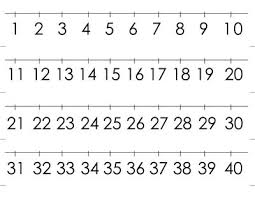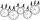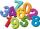# Three-digit integers

How many three-digit natural numbers exist that do not contain zero and are divisible by five?

n =  81

### Step-by-step explanation:Did you find an error or inaccuracy? Feel free to write us. Thank you!Tips to related online calculators
Would you like to compute count of combinations?

## Related math problems and questions:

• Three-digit numbersHow many are all three-digit numbers that are made up of digits 0,2,5,7 and are divisible by 9 if the digits can be repeated?
• Three digits numberHow many are three-digit integers such that they no digit repeats?
• Three digits numberFrom the numbers 1, 2, 3, 4, 5 create three-digit numbers that digits not repeat and number is divisible by 2. How many numbers are there?
• A three-digit numbersDetermine the total number of positive three-digit numbers that contain a digit 6.
• Three-digitHow many do three-digit natural numbers not have the number 7?
• How many 4How many four-digit numbers that are divisible by ten can be created from the numbers 3, 5, 7, 8, 9, 0 such no digits repeats?
• Five-digit numberAnna thinks of a five-digit number that is not divisible by three or four. If he increments each digit by one, it gets a five-digit number that is divisible by three. If he reduces each digit by one, he gets a five-digit number divisible by four. If it sw
• Twenty-fiveHow many are three-digit natural numbers divisible by 25?
• Divisible by fiveHow many different three-digit numbers divisible by five can we create from the digits 2, 4, 5? We can repeat the digits in the created number.
• Three-digit numbersUse the numbers 4,5,8,9 to write all three-digit numbers without repetition. How many such numbers are there?
• CombinationsHow many different combinations of two-digit number divisible by 4 arises from the digits 3, 5 and 7?
• Air draftThe numbers 1,2,3,4,5 are written on five tickets on the table. Air draft randomly shuffled the tickets and composed a 5-digit number from them. What is the probability that he passed: and, the largest possible number b, the smallest possible number c, a
• ID tagHow many 3 digit ID tag can be made if: a) a digit cannot be used more than once b) the  1st digit must be five, and repetition of the others is not permitted.
• Three-digit numbersHow many three-digit numbers are from the numbers 0 2 4 6 8 (with/without repetition)?
• Inverted nineIn the hotel Inverted Nine, each hotel room number is divisible by 6. How many rooms can we count with the three-digit number registered by digits 1,8,7,4,9?
• Three-digitHow many three-digit natural numbers are greater than 321 if no digit in number repeated?
• Divisible by 5How many three-digit odd numbers divisible by 5, which are in place ten's number 3?